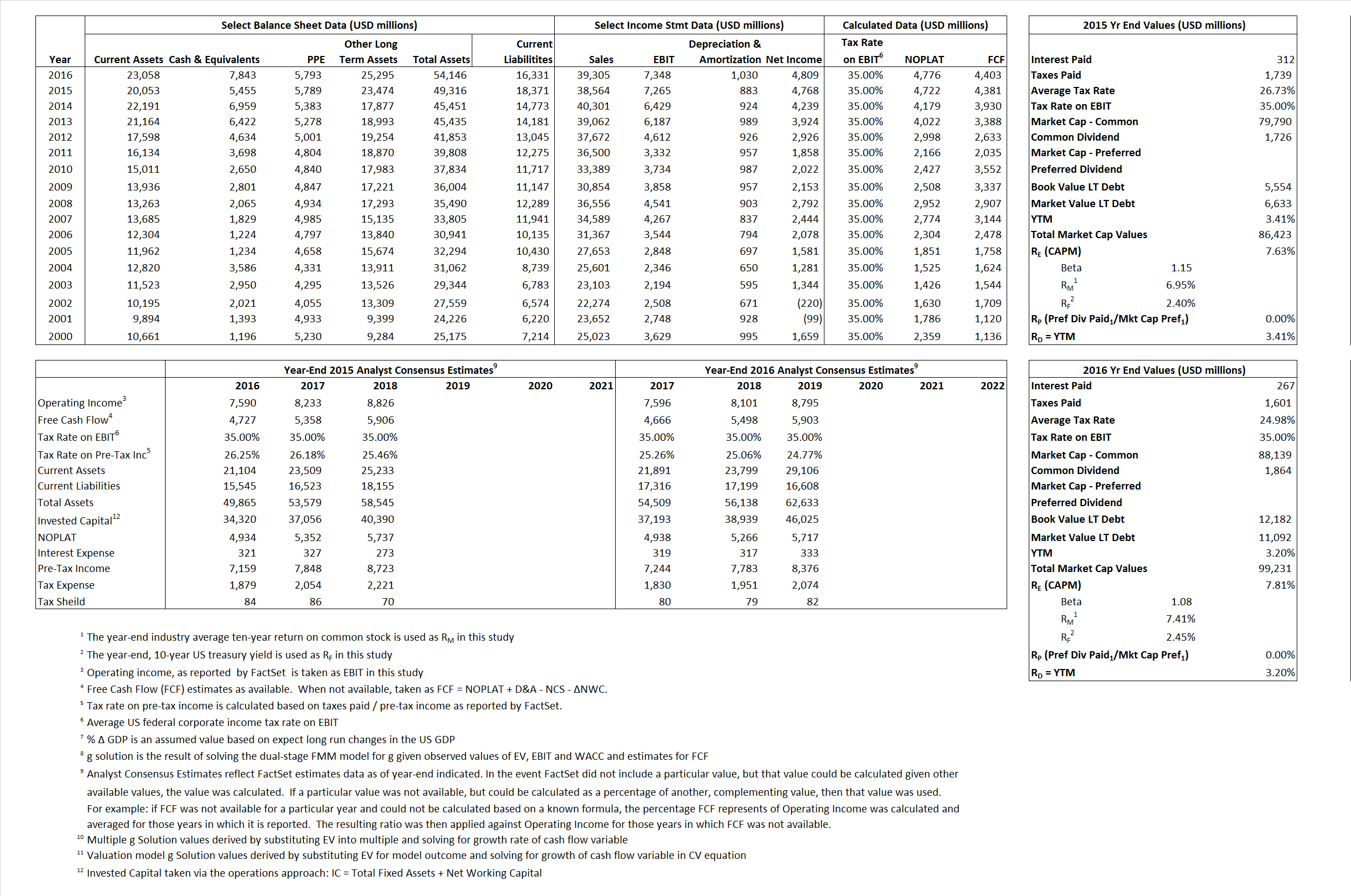# Honeywell

## Analyst Listing

The following analysts provide coverage for the subject firm as of May 2016:

 Broker Analyst Analyst Email Morningstar Barbara Noverini barbara.noverini@morningstar.com Langenberg, LLC Brian K. Langenberg brian@langenberg-llc.com Oppenheimer Christopher Glynn christopher.glynn@opco.com RBC Capital Markets Deane M. Dray deane.dray@rbccm.com Cowen & Company Gautam Khanna gautam.khanna@cowen.com Jefferies Howard A. Rubel hrubel@jefferies.com Gabelli & Company James Foung jfoung@gabelli.com Argus Research John Eade jeade@argusresearch.com Deutsche Bank Research John G. Inch john.inch@db.com Credit Suisse Julian Mitchell julian.mitchell@credit-suisse.com William Blair Nicholas P. Heymann nheymann@williamblair.com Sterne Agee CRT Peter Arment parment@sterneageecrt.com Atlantic Equities Richard Radbourne r.radbourne@atlantic-equities.com Drexel Hamilton Richard Whittington rwhittington@drexelhamilton.com Stifel Nicolaus Robert P. McCarthy mccarthyr@stifel.com Bernstein Research Steven E. Winoker steven.winoker@bernstein.com

## Primary Input Data## Derived Input Data

### Equational Form

Net Operating Profit Less Adjusted Taxes NOPLAT 4,722 4,776$NOPLAT\, =\, EBIT\, x\, (1 \,-\, Avg \,\,Tax\,\, Rate\,\, on\,\, EBIT)$
Free Cash Flow FCF 4,381 4,403$FCF\,=NOPLAT\,+\,Non-Cash\,Expenses-\Delta NWC\,-\,NCS$
Tax Shield TS 83  67$TS\,=\,Interest\,\,Paid\,\,x\,\, Avg \,\,Tax\,\,Rate\,\, on\,\, Pre-Tax\,\, Income$
Invested Capital IC 30,945 37,815$IC\,=\,Fixed\,\,Operating\,\,Assets\,\,+\,\,Net\,\, Working\,\, Capital$
Return on Invested Capital ROIC 15.26% 12.63%$ROIC\,=\,\frac { NOPLAT }{ IC }$
Net Investment NetInv  1,150  7,900$NetInv\,=\,{ {IC}_{1}}-{{IC}_{0}}+Depreciation$
Investment Rate IR  24.35% 165.40%$IR\,=\,\frac {NetInv}{NOPLAT}$
Weighted Average Cost of Capital
WACCMarket  7.24%  7.20%$WACC\,=\,\frac { E }{ V } { R }_{ E }\,+\,\frac { P }{ V } { R }_{ P }\,+\,\frac { D }{ V } { R }_{ D }\left( 1- Avg\,\, Tax\,\,Rate\,\,on\,\,Pre-Tax\,\,Income \right)$
WACCBook   9.31%  8.38%
Enterprise value
EVMarket  80,968  91,388$EV\,=\,Market\,\,Cap\,\,Equity\,+\,\,Long\,\,Term\,\,Debt\,-\,Cash$
EVBook  79,889   92,478
Long-Run Growth
g = IR x ROIC
3.72%   20.89% Long-run growth rates of the income variable are used in the Continuing Value portion of the valuation models.
g = %$\Delta$ GDP   2.50%    2.50%
Margin from Operations M  18.84%  18.69%$M\,\,=\,\,\frac{EBIT}{SALES}$
Depreciation/Amortization Rate D  10.84%  12.29%$D\,\,=\,\,\frac{D+A}{EBITDA}$

## Valuation Multiple Outcomes

The outcomes presented in this study are the result of original input data, derived data, and synthesized inputs.

### model g solution

12/31/2015 12/31/2016 12/31/2015 12/31/2016 12/31/2015 12/31/2016

EV/SALES$\frac {EV}{Sales} \,= \,\frac{ROIC\, -\, g}{ROIC\,(WACC\,-\,g)}\,(1\,-\,T)\,(M)$

2.10  2.33  2.28%  3.37%  2.45%  4.46%

EV/EBITDA$\frac {EV}{EBITDA} \,= \,\frac{ROIC\, -\, g}{ROIC\,(WACC\,-\,g)}\,(1\,-\,T)\,(1\,-\,D)$

9.94 10.91 2.28% 3.37% 2.45% 4.46%

EV/NOPLAT$\frac {EV}{NOPLAT} \,= \,\frac{ROIC\, -\, g}{ROIC\,(WACC\,-\,g)}$

17.25 19.13 2.28% 3.37% 2.45% 4.46%

EV/FCFOPS$\frac {EV}{FCF_{OPS}} \,= \,\frac{ROIC\, -\, g}{ROIC\,(WACC\,-\,g)}\,(1\,-\,T)$

18.48 20.76 2.28% 3.37% 2.45% 4.46%

EV/EBIT$\frac {EV}{EBIT} \,= \,\frac{ROIC\, -\, g}{ROIC\,(WACC\,-\,g)}\,(1\,-\,T)$

11.14 12.44 2.28% 3.37% 2.45% 4.46%

EV/IC$\frac {EV}{IC} \,= \,\frac{ROIC\, -\, g}{WACC\,-\,g}$

2.62 2.42 2.28% 3.37% 2.45% 4.46%